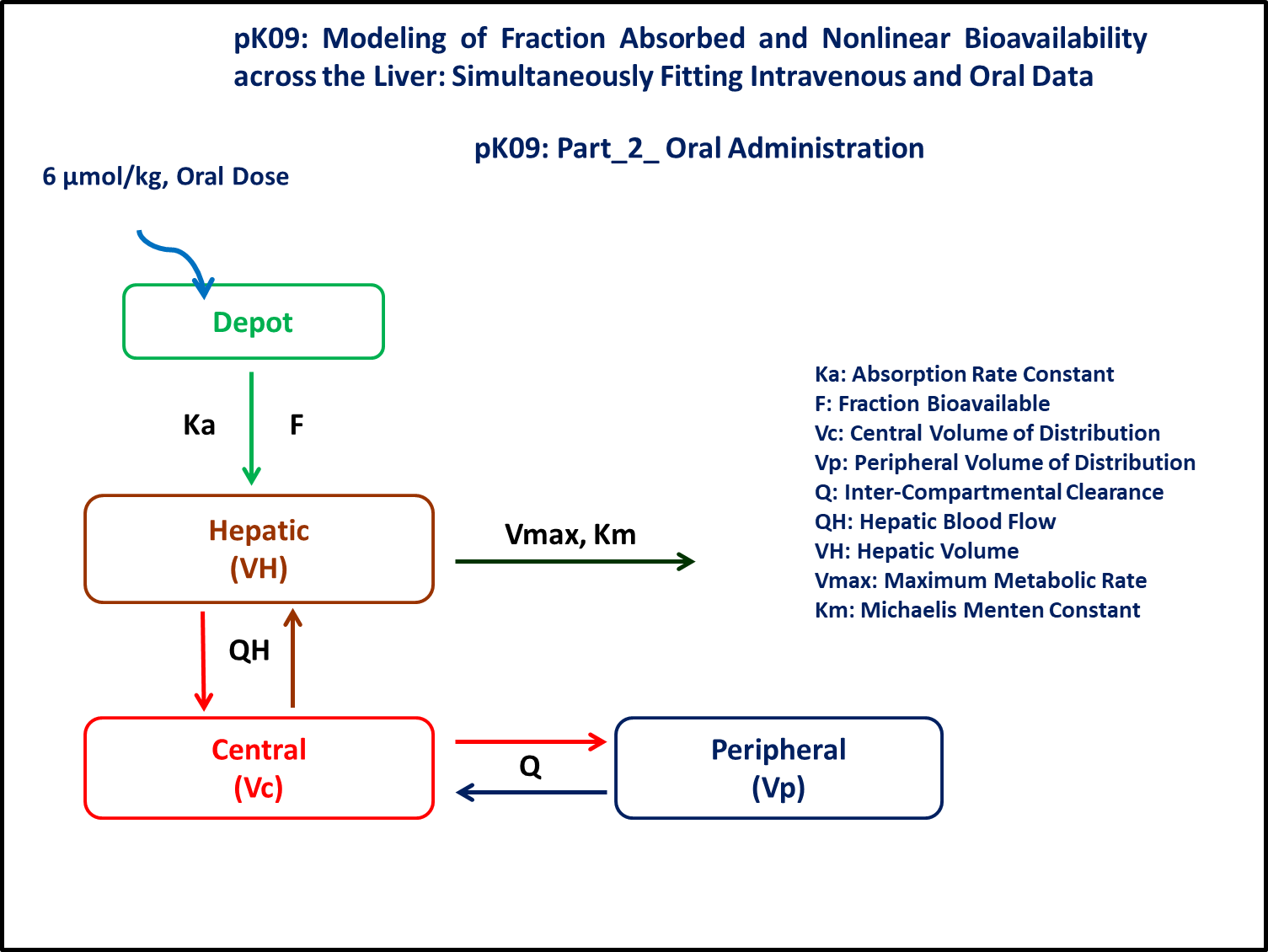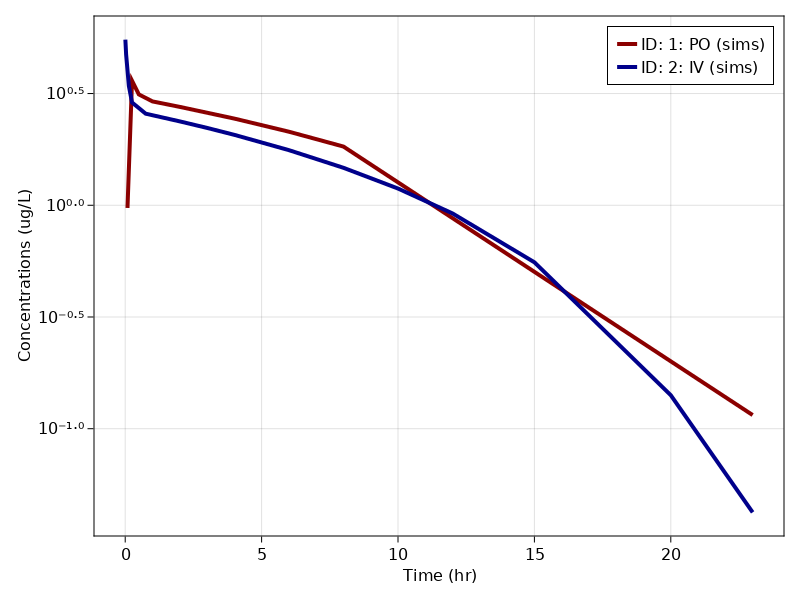# Exercise PK09 - Modeling of fraction absorbed and nonlinear bioavailability across the liver, Simulataneous fitting of Intravenous and Oral data

### Background

• Structural model - Two compartment model with non-linear elimination from hepatic compartment

• Route of administration - Oral and IV on separate occasion

• Dosage Regimen - 2 μmol/kg : IV and 6 μmol/kg : Oral

• Number of Subjects - 1### Learning Outcomes

Semi-physiologic model with elimination from hepatic compartment and dose administered by IV bolus. The drug follows a two compartment model. This data was modeled taking into account the hepatic elimination component and hepatic blood flow. Hepatic distribution and elimination from liver is modeled as an additional compartment with physiological values of blood flow reported from literature. Oral dose administered into a Depot. This Depot compartment was connected to the liver compartment.This study was conducted with an Oral suspension of 6 μmol/kg and IV bolus of 2 μmol/kg.

### Objective

The exercise aims to simulate data using a two compartment model and an additional hepatic compartment. The elimination is non-linear metabolic clearance from the liver. In case of oral administration the administered dose reaches hepatic compartment with a lag-time.

### Libraries

Call the "necessary" libraries to get started

using Random
using Pumas
using PumasUtilities
using CairoMakie


### Model

This model is written for both Oral and IV dosing regimen

pk_09    = @model begin
desc = "Semi-Physiologic Model"
timeu = u"hr"
end

@param begin
"Volume of Central Compartment (L/kg)"
tvvc        ∈ RealDomain(lower=0)
"Inter-compartmental Clearance (L/hr/kg)"
tvq         ∈ RealDomain(lower=0)
"Volume of Peripheral Compartment (L/kg)"
tvvp        ∈ RealDomain(lower=0)
"Maximum Metabolic Rate (μmol/hr/kg)"
tvvmax      ∈ RealDomain(lower=0)
"Michaelis Menton Constant (μmol/L)"
tvkm        ∈ RealDomain(lower=0)
"Absorption Rate Constant (hr⁻¹)"
tvka        ∈ RealDomain(lower=0)
"Lag-time (hr)"
tvtlag      ∈ RealDomain(lower=0)
"Fraction of drug absorbed"
tvfa        ∈ RealDomain(lower=0)
Ω           ∈ PDiagDomain(8)
"Proportional RUV"
σ²_prop     ∈ RealDomain(lower=0)
end

@random begin
η           ~ MvNormal(Ω)
end

@pre begin
Vc          = tvvc * exp(η)
Q           = tvq * exp(η)
Vp          = tvvp * exp(η)
Vmax        = tvvmax * exp(η)
Km          = tvkm * exp(η)
Ka          = tvka * exp(η)
Qh          = 3.3
Vh          = 0.02
end

@dosecontrol begin
lags        = (Depot = tvtlag * exp(η),)
bioav       = (Depot = tvfa * exp(η),)
end

@vars begin
VMKM       := Vmax*(Hepatic/Vh)/(Km + (Hepatic/Vh))
end

@dynamics begin
Depot'      = - Ka*Depot
Hepatic'    =   Ka*Depot -(Qh/Vh)*Hepatic + (Qh/Vc)*Central - VMKM
Central'    =  (Qh/Vh)*Hepatic - (Qh/Vc)*Central -(Q/Vc)*Central + (Q/Vp)*Peripheral
Peripheral' =  (Q/Vc)*Central - (Q/Vp)*Peripheral
end

@derived begin
cp          = @. Central/Vc
"""
Observed Concentration (ug/L)
"""
dv          ~ @. Normal(cp, sqrt(cp^2*σ²_prop))
end
end

PumasModel
Parameters: tvvc, tvq, tvvp, tvvmax, tvkm, tvka, tvtlag, tvfa, Ω, σ²_prop
Random effects: η
Covariates:
Dynamical variables: Depot, Hepatic, Central, Peripheral
Derived: cp, dv
Observed: cp, dv


### Parameters

Parameters provided for simulation. tv represents the typical value for parameters.

• $Vc$ - Volume of Central Compartment (L/kg)

• $Q$ - Intercompartmental Clearance (L/kg)

• $Vp$ - Volume of Peripheral Compartment (L/kg)

• $Vmax$ - Maximum Metabolic Rate (μmol/hr/kg)

• $Km$ - Michaelis Menton Constant (μmol/L)

• $Ka$ - Absorption Rate Constant (hr⁻¹)

• $fa$ - Fraction of drug absorbed

• $tlag$ - lag time (hr)

param = (tvvc    = 0.34,
tvq     = 1.84,
tvvp    = 0.38,
tvvmax  = 0.13,
tvkm    = 0.31,
tvka    = 11.3,
tvfa    = 0.38,
tvtlag  = 0.062,
Ω       = Diagonal([0.0,0.0,0.0,0.0,0.0,0.0,0.0,0.0]),
σ²_prop = 0.00)

(tvvc = 0.34, tvq = 1.84, tvvp = 0.38, tvvmax = 0.13, tvkm = 0.31, tvka = 1
1.3, tvfa = 0.38, tvtlag = 0.062, Ω = [0.0 0.0 … 0.0 0.0; 0.0 0.0 … 0.0 0.0
; … ; 0.0 0.0 … 0.0 0.0; 0.0 0.0 … 0.0 0.0], σ²_prop = 0.0)


### Dosage Regimen

##### Oral

Dose of 6 μmol/kg is administered orally at time=0

ev1  = DosageRegimen(6, time = 0, cmt = :Depot)
sub1 = Subject(id = "1: PO", events = ev1)

Subject
ID: 1: PO
Events: 1

##### IV

Dose of 2 μmol/kg is administered as IV-bolus at time=0

ev2  = DosageRegimen(2, time = 0, cmt = :Central)
sub2 = Subject(id = "2: IV", events = ev2)

Subject
ID: 2: IV
Events: 1


### Simulation

##### Oral
Random.seed!(123)
sim_sub1_oral = simobs(pk_09, sub1, param,
obstimes = [0.08333,0.25,0.5,1,2,4,6,8,23])

##### IV
Random.seed!(123)
sim_sub2_iv = simobs(pk_09, sub2, param,
obstimes = [0.0056,0.03333,0.13333,0.25,0.75,1,2,3,4,6,8,10,12,15,20,23])


### Visualization

f, a, p = sim_plot(pk_09, [sim_sub1_oral, sim_sub2_iv],
observations=:cp,
color = :redsblues, linewidth = 4,
axis = (yscale = log10,
xlabel = "Time (hr)",
ylabel = "Concentrations (ug/L)",
xticks = 0:5:25))
axislegend(a)
f